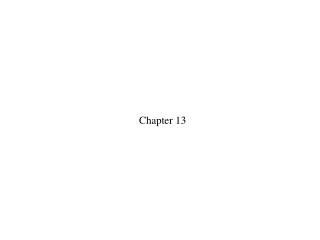DownloadDownload PresentationChapter 13

# Chapter 13

Télécharger la présentation## Chapter 13

- - - - - - - - - - - - - - - - - - - - - - - - - - - E N D - - - - - - - - - - - - - - - - - - - - - - - - - - -
##### Presentation Transcript

1. Chapter 13

2. The fan blade is slowing down. What are the signs of 1. 2. 3. 4.

3. The fan blade is slowing down. What are the signs of 1. 2. 3. 4.

4. Rank in order, from largest to smallest, the five torques The rods all have the same length and are pivoted at the dot. 1. 2. 3. 4. 5.

5. Rank in order, from largest to smallest, the five torques The rods all have the same length and are pivoted at the dot. 1. 2. 3. 4. 5.

6. Rank in order, from largest to smallest, the angular accelerations 1. 2. 3. 4. 5.

7. Rank in order, from largest to smallest, the angular accelerations 1. 2. 3. 4. 5.

8. Four Ts are made from two identical rods of equal mass and length. Rank in order, from largest to smallest, the moments of inertia Ia to Id for rotation about the dotted line. 1. Ic > Ib > Id > Ia 2. Ic = Id > Ia = Ib 3. Ia = Ib > Ic = Id 4. Ia > Id > Ib > Ic 5. Ia > Ib > Id > Ic

9. Four Ts are made from two identical rods of equal mass and length. Rank in order, from largest to smallest, the moments of inertia Ia to Id for rotation about the dotted line. 1. Ic > Ib > Id > Ia 2. Ic = Id > Ia = Ib 3. Ia = Ib > Ic = Id 4. Ia > Id > Ib > Ic 5. Ia > Ib > Id > Ic

10. A student holds a meter stick straight out with one or more masses dangling from it. Rank in order, from most difficult to least difficult, how hard it will be for the student to keep the meter stick from rotating. 1. c > d > b > a 2. b = c = d > a 3. c > b > d > a 4. b > d > c > a 5. c > d > a = b

11. A student holds a meter stick straight out with one or more masses dangling from it. Rank in order, from most difficult to least difficult, how hard it will be for the student to keep the meter stick from rotating. 1. c > d > b > a 2. b = c = d > a 3. c > b > d > a 4. b > d > c > a 5. c > d > a = b

12. Two buckets spin around in a horizontal circle on frictionless bearings. Suddenly, it starts to rain. As a result, 1. The buckets slow down because the angular momentum of the bucket + rain system is conserved. 2. The buckets continue to rotate at constant angular velocity because the rain is falling vertically while the buckets move in a horizontal plane. 3. The buckets continue to rotate at constant angular velocity because the total mechanical energy of the bucket + rain system is conserved. 4. The buckets speed up because the potential energy of the rain is transformed into kinetic energy. 5. None of the above.

13. Two buckets spin around in a horizontal circle on frictionless bearings. Suddenly, it starts to rain. As a result, 1. The buckets slow down because the angular momentum of the bucket + rain system is conserved. 2. The buckets continue to rotate at constant angular velocity because the rain is falling vertically while the buckets move in a horizontal plane. 3. The buckets continue to rotate at constant angular velocity because the total mechanical energy of the bucket + rain system is conserved. 4. The buckets speed up because the potential energy of the rain is transformed into kinetic energy. 5. None of the above.